# 7.3 使用TensorBoard可视化训练过程#

TensorBoard作为一款可视化工具能够满足上面提到的各种需求。TensorBoard由TensorFlow团队开发，最早和TensorFlow配合使用，后来广泛应用于各种深度学习框架的可视化中来。本节我们探索TensorBoard的强大功能，希望帮助读者“从入门到精通”。

• 安装TensorBoard工具

• 了解TensorBoard可视化的基本逻辑

• 掌握利用TensorBoard实现训练过程可视化

• 掌握利用TensorBoard完成其他内容的可视化

## 7.3.1 TensorBoard安装#

pip install tensorboardX


## 7.3.3 TensorBoard的配置与启动#

from tensorboardX import SummaryWriter

writer = SummaryWriter('./runs')


from torch.utils.tensorboard import SummaryWriter


tensorboard --logdir=/path/to/logs/ --port=xxxx


## 7.3.4 TensorBoard模型结构可视化#

import torch.nn as nn

class Net(nn.Module):
def __init__(self):
super(Net, self).__init__()
self.conv1 = nn.Conv2d(in_channels=3,out_channels=32,kernel_size = 3)
self.pool = nn.MaxPool2d(kernel_size = 2,stride = 2)
self.conv2 = nn.Conv2d(in_channels=32,out_channels=64,kernel_size = 5)
self.flatten = nn.Flatten()
self.linear1 = nn.Linear(64,32)
self.relu = nn.ReLU()
self.linear2 = nn.Linear(32,1)
self.sigmoid = nn.Sigmoid()

def forward(self,x):
x = self.conv1(x)
x = self.pool(x)
x = self.conv2(x)
x = self.pool(x)
x = self.flatten(x)
x = self.linear1(x)
x = self.relu(x)
x = self.linear2(x)
y = self.sigmoid(x)
return y

model = Net()
print(model)


Net(
(conv1): Conv2d(3, 32, kernel_size=(3, 3), stride=(1, 1))
(pool): MaxPool2d(kernel_size=2, stride=2, padding=0, dilation=1, ceil_mode=False)
(conv2): Conv2d(32, 64, kernel_size=(5, 5), stride=(1, 1))
(flatten): Flatten(start_dim=1, end_dim=-1)
(linear1): Linear(in_features=64, out_features=32, bias=True)
(relu): ReLU()
(linear2): Linear(in_features=32, out_features=1, bias=True)
(sigmoid): Sigmoid()
)


writer.add_graph(model, input_to_model = torch.rand(1, 3, 224, 224))
writer.close()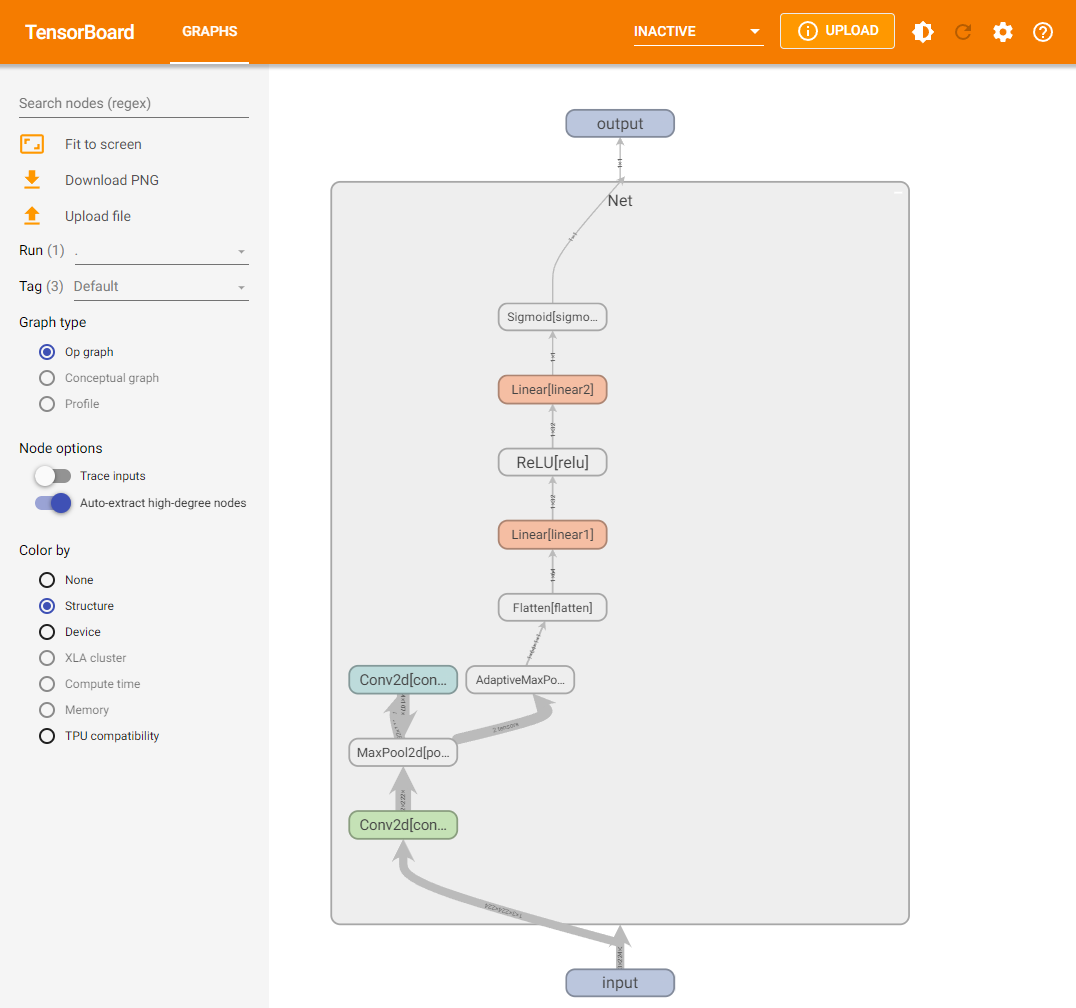## 7.3.5 TensorBoard图像可视化#

import torchvision
from torchvision import datasets, transforms

transform_train = transforms.Compose(
[transforms.ToTensor()])
transform_test = transforms.Compose(
[transforms.ToTensor()])

# 仅查看一张图片
writer = SummaryWriter('./pytorch_tb')
writer.close()

# 将多张图片拼接成一张图片，中间用黑色网格分割
# create grid of images
writer = SummaryWriter('./pytorch_tb')
img_grid = torchvision.utils.make_grid(images)
writer.close()

# 将多张图片直接写入
writer = SummaryWriter('./pytorch_tb')
writer.close()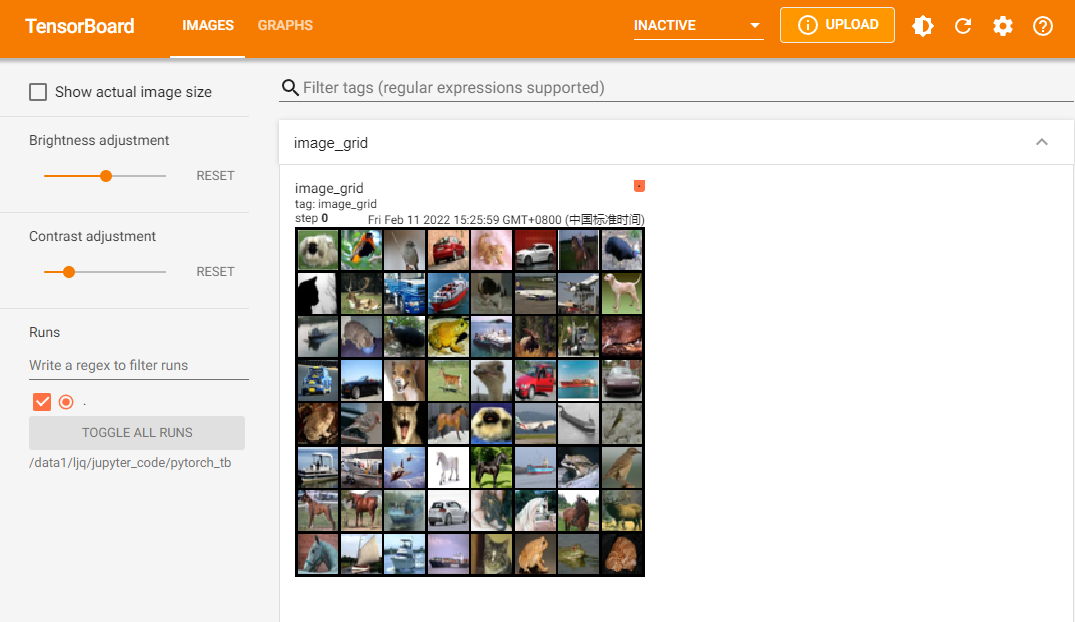## 7.3.6 TensorBoard连续变量可视化#

writer = SummaryWriter('./pytorch_tb')
for i in range(500):
x = i
y = x**2
writer.add_scalar("x", x, i) #日志中记录x在第step i 的值
writer.add_scalar("y", y, i) #日志中记录y在第step i 的值
writer.close()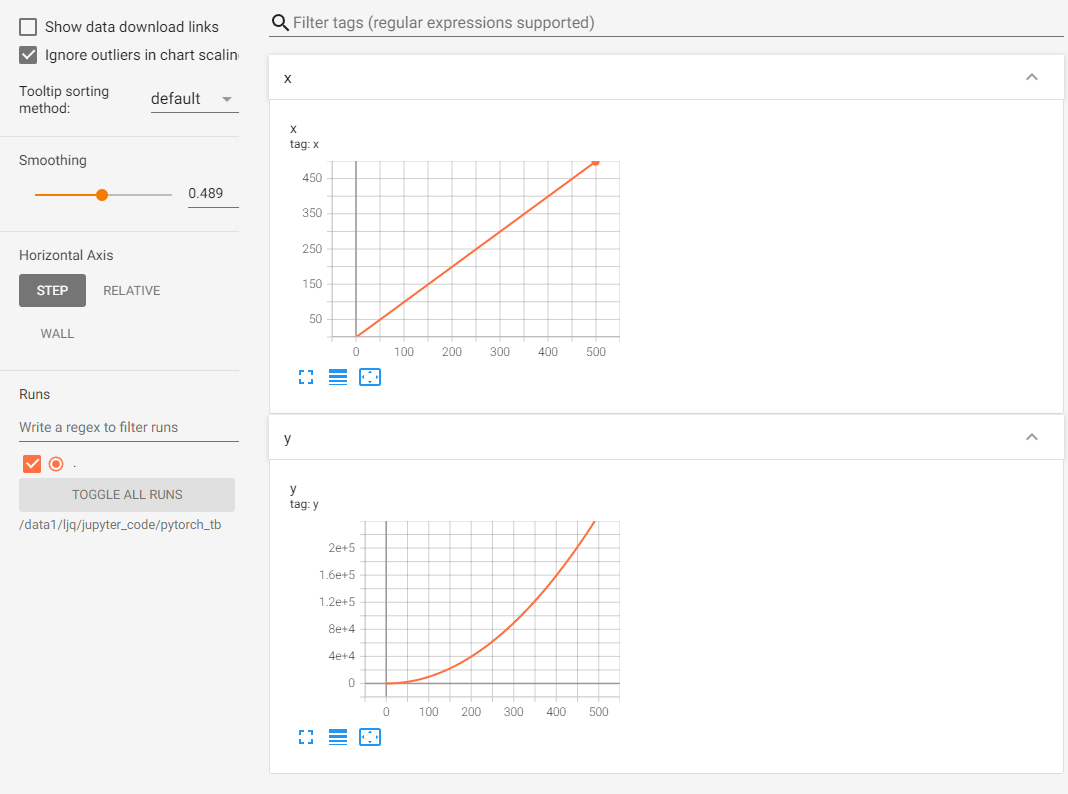writer1 = SummaryWriter('./pytorch_tb/x')
writer2 = SummaryWriter('./pytorch_tb/y')
for i in range(500):
x = i
y = x*2
writer1.add_scalar("same", x, i) #日志中记录x在第step i 的值
writer2.add_scalar("same", y, i) #日志中记录y在第step i 的值
writer1.close()
writer2.close()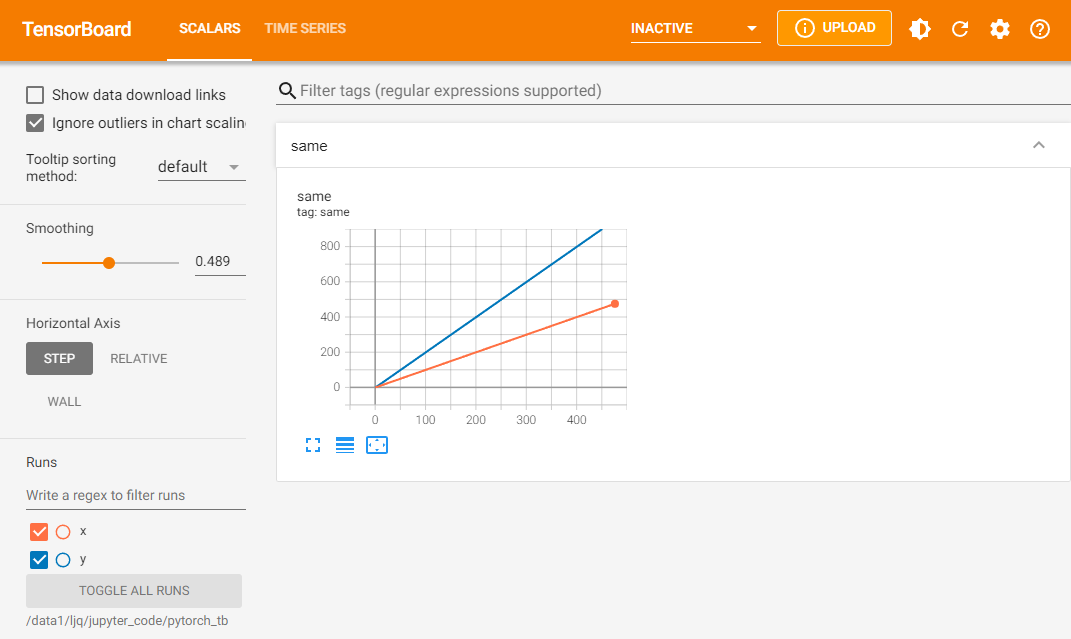## 7.3.7 TensorBoard参数分布可视化#

import torch
import numpy as np

# 创建正态分布的张量模拟参数矩阵
def norm(mean, std):
t = std * torch.randn((100, 20)) + mean
return t

writer = SummaryWriter('./pytorch_tb/')
for step, mean in enumerate(range(-10, 10, 1)):
w = norm(mean, 1)
writer.flush()
writer.close()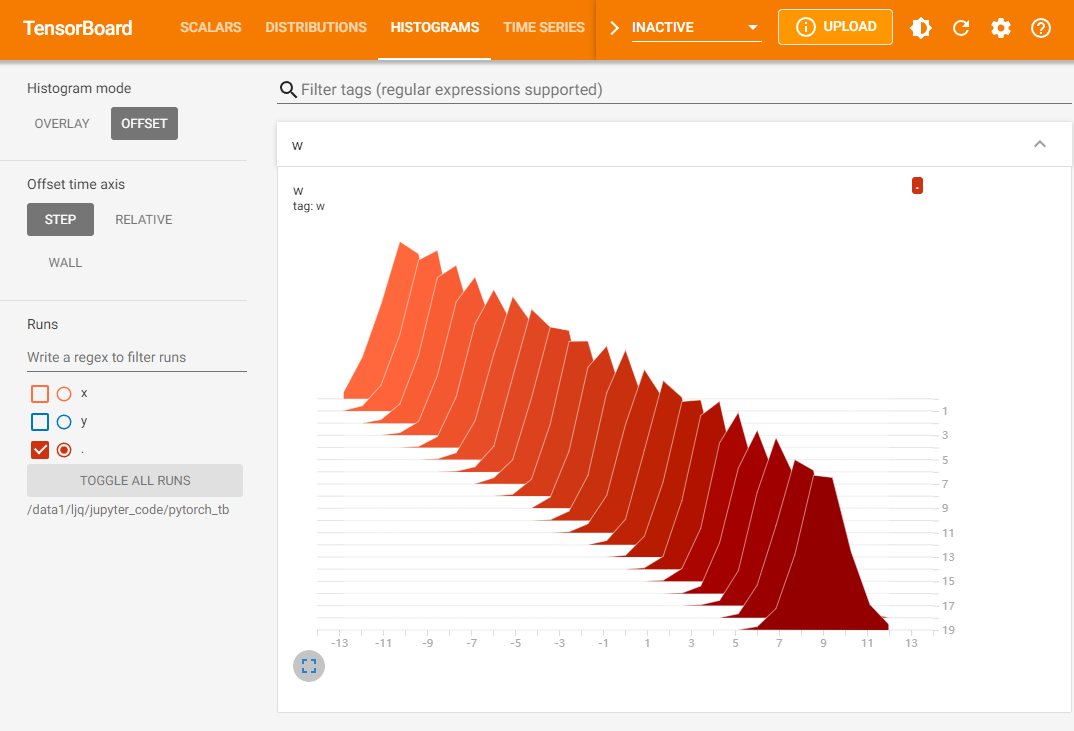## 7.3.8 服务器端使用TensorBoard#

• MobaXterm

1. 在MobaXterm点击Tunneling

2. 选择New SSH tunnel，我们会出现以下界面。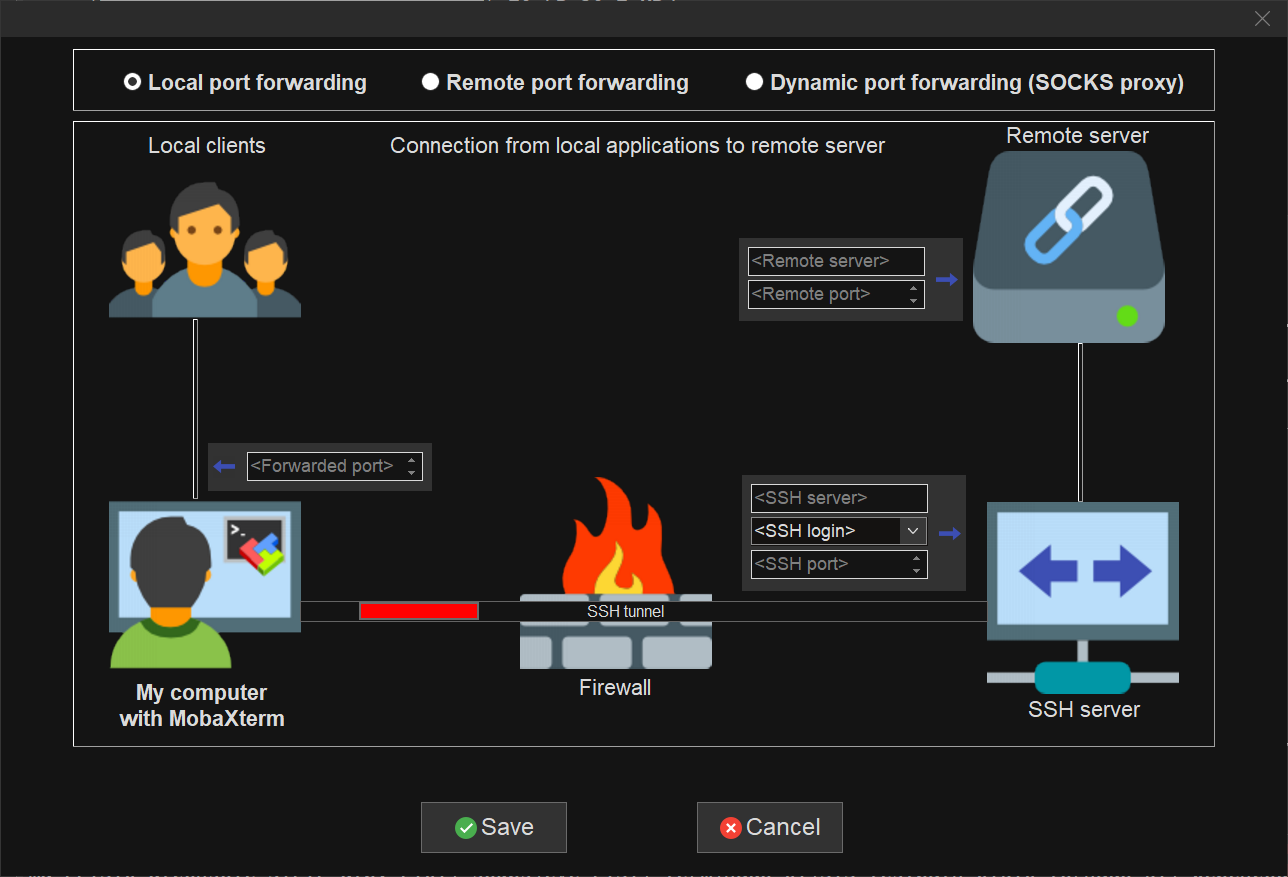1. 对新建的SSH通道做以下设置，第一栏我们选择Local port forwarding< Remote Server>我们填写localhost< Remote port>填写6006，tensorboard默认会在6006端口进行显示，我们也可以根据 tensorboard --logdir=/path/to/logs/ --port=xxxx的命令中的port进行修改，< SSH server> 填写我们连接服务器的ip地址，<SSH login>填写我们连接的服务器的用户名，<SSH port>填写端口号（通常为22），< forwarded port>填写的是本地的一个端口号，以便我们后面可以对其进行访问。

2. 设定好之后，点击Save，然后Start。在启动tensorboard，这样我们就可以在本地的浏览器输入http://localhost:6006/对其进行访问了

• Xshell

1. Xshell的连接方法与MobaXterm的连接方式本质上是一样的，具体操作如下：

2. 连接上服务器后，打开当前会话属性，会出现下图，我们选择隧道，点击添加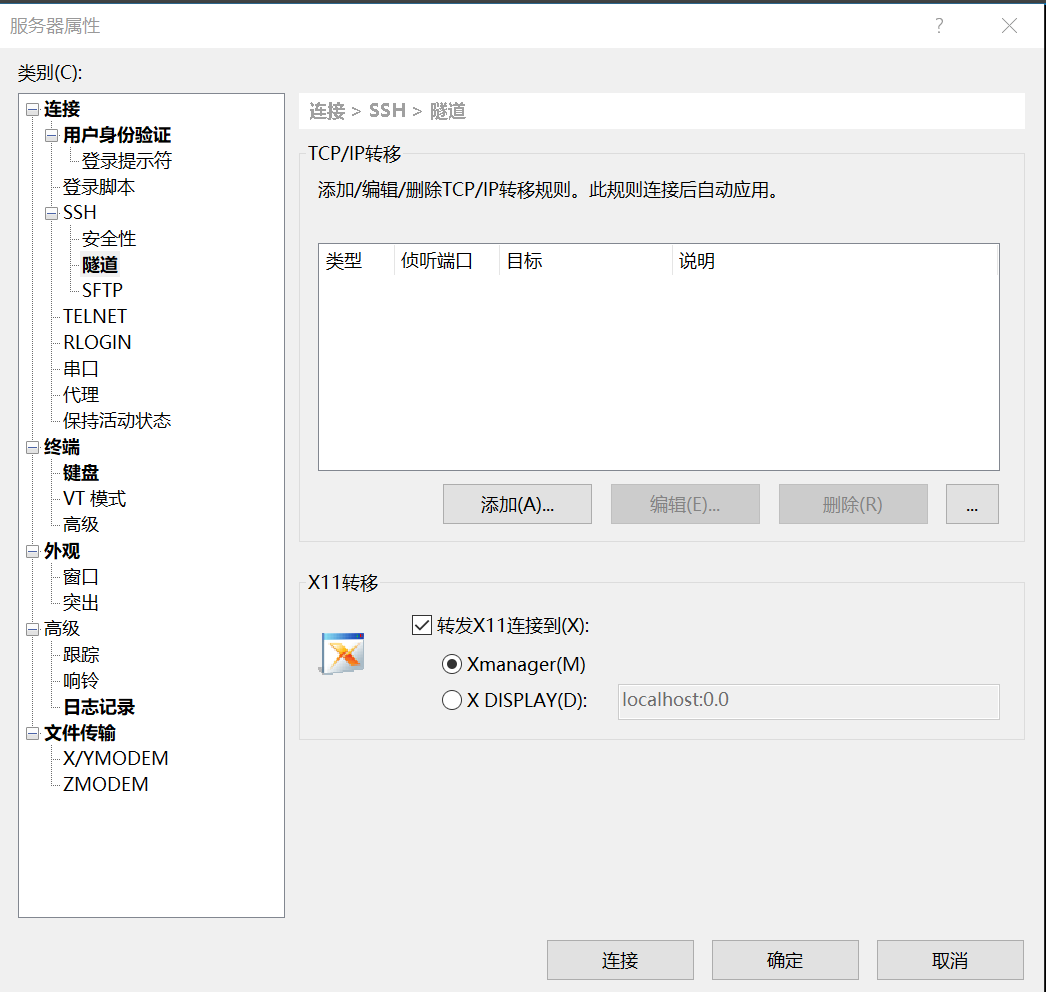3. 按照下方图进行选择，其中目标主机代表的是服务器，源主机代表的是本地，端口的选择根据实际情况而定。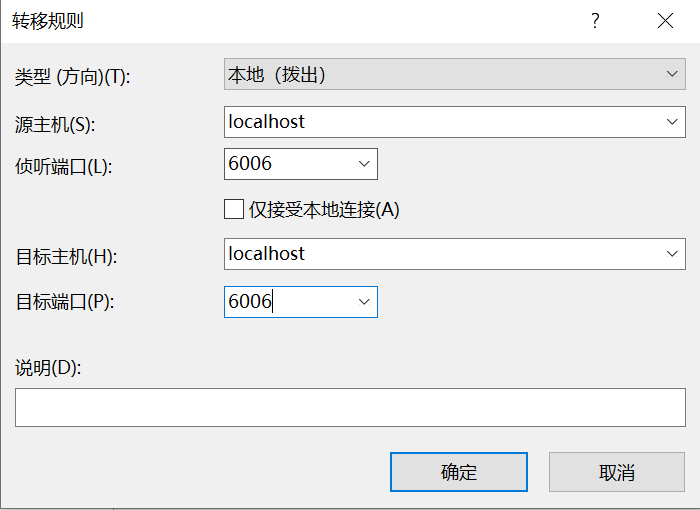4. 启动tensorboard，在本地127.0.0.1:6006 或者 localhost:6006进行访问。

• SSH

1. 该方法是将服务器的6006端口重定向到自己机器上来，我们可以在本地的终端里输入以下代码：其中16006代表映射到本地的端口，6006代表的是服务器上的端口。

  ssh -L 16006:127.0.0.1:6006 username@remote_server_ip

1. 在服务上使用默认的6006端口正常启动tensorboard

tensorboard --logdir=xxx --port=6006

1. 在本地的浏览器输入地址

127.0.0.1:16006 或者 localhost:16006